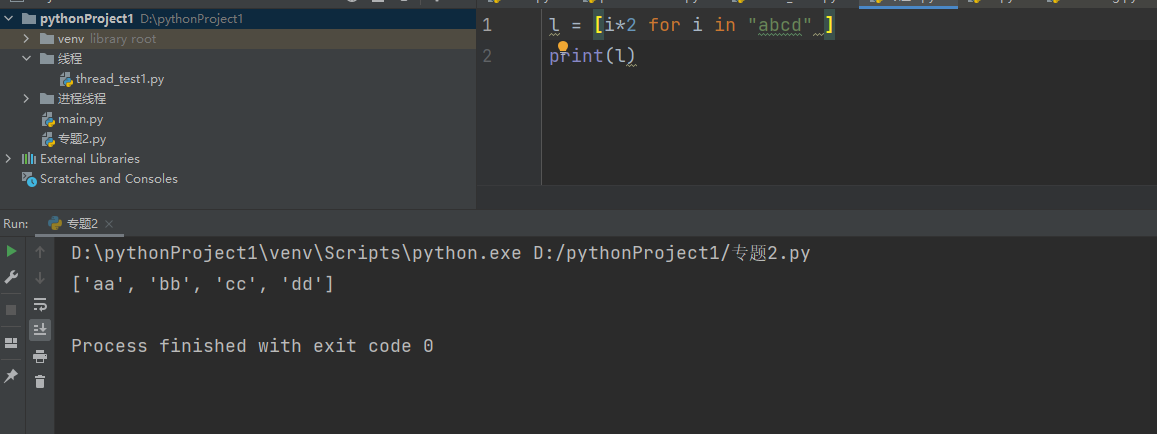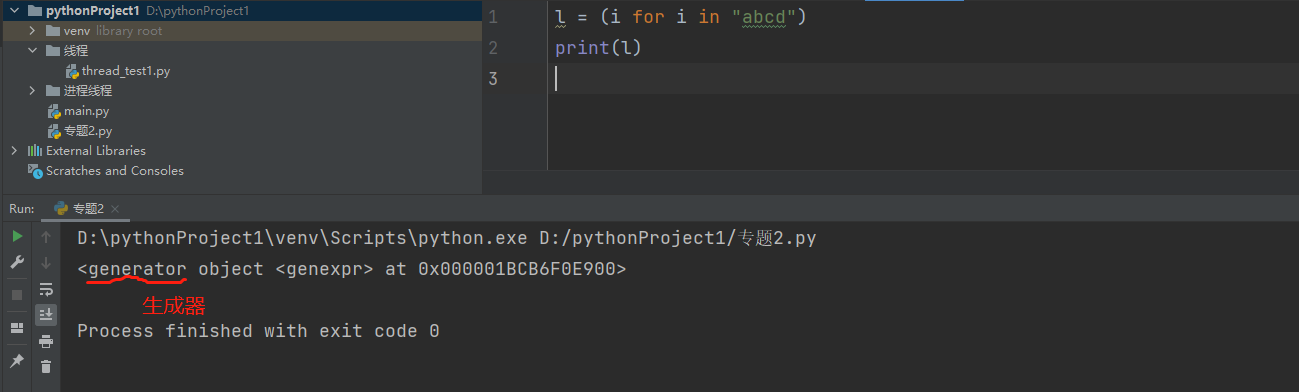﻿ 稳扎稳打学Python之容器 可迭代对象 迭代器 生成器专题讲解_python_脚本之家
python# 稳扎稳打学Python之容器 可迭代对象 迭代器 生成器专题讲解## 一、容器

### 1.什么是容器？## 二、可迭代对象

### 1.什么是可迭代对象？

• 1.很多容器都是可迭代对象（容器包含了可迭代对象）。
• 2.一个可迭代对象是不能独立的进行迭代的，迭代是通过for来完成的，凡是可迭代对象都可以直接使用for循环进行访问。

for循环大家应该不陌生吧？有没有想过，for循环内部是怎么实现的？比如说这个for循环的例子，为什么能输出列表里的每一个元素？它的内部是怎么实现的？• 1.使用 __iter__() 返回1个迭代器，迭代器在下面会讲，这里先知道有这么个东西。
• 2.使用 __next__() 获取迭代器中的每一个元素。

```l = [1,2,3,4]
# for i in l:
#     print(i)
ite =l.__iter__() #接收一下ietr()干了什么
print(ite)  #打印
print(ite.__next__())    #for循环干第2件事情的时候做的第1步
print(ite.__next__())    #for循环干第2件事情的时候做的第2步
print(ite.__next__())    #for循环干第2件事情的时候做的第3步
print(ite.__next__())    #for循环干第2件事情的时候做的第4步
```• 内置函数 iter() 的本质是 __inter__() ，也是返回一个迭代器。
• 内置函数 next（） 的本质是 __next__()，也是有了迭代器之后获取元素。## 三、迭代器

• 只要是实现了__iter__()和__next__()的对象，就是迭代器，迭代器是一个可迭代对象。
• 总之，迭代器是有__iter__()生成，可以通过__next__()进行调用。## 四、序列## 五、列表推导式

```l = [i for i in 可迭代对象]
```

i表示要放进列表里的对象，for循环是一个式子。

```l = [i for i in range(5)]
print(l)
```

[0, 1, 2, 3, 4]

```l = [i for i in range(5) if 4<5]
print(l)
```

[0, 1, 2, 3, 4]

if后面的条件判断为真，则可以正常生成列表，如果为假，则列表推导式是无效的，此时的l将是一个空列表。[] 换成（）之后，返回的是一个生成器generrator ，那么下面我们再来讲讲生成器：

## 六、生成器

### 1.生成器的第一种创建方法：生成器表达式

```l = (i for i in 可迭代对象)
```

```l = (i for i in "abcd")
print(next(l))
```

a

### 2.生成器的第二种创建方法：yield

yield 关键字

```def fun():
a = 10
while 1:
a += 1
yield a

b = fun()
print(b)
```

<generator object fun at 0x000001F2AD95E900>

```def fun():
a = 10
while 1:
a += 1
yield a

b = fun()
print(next(b))
print(next(b))
print(next(b))
```

11
12
13

```def fun():
a = 10
while 1:
a += 1
print(a)

b = fun()
```## 七、小结